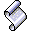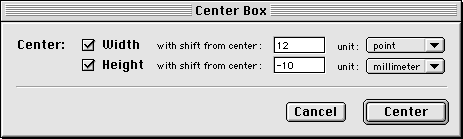Previous  |  Main  |  Next#### Center Boxes

Script for: QuarkXPress
Description: Script A:
Centers a single or multiple selected boxes either by width or by height.
Optionally a shift from this center may be specified in one of the following units:
picas, points, inches, centimeters or millimeters.

Script B:
Enhanced version featuring a customized dialog (as shown below).
Differences from Script A:
• The custom dialog is easier to use and provides some error checking.
• You may center the selected box(es) by width and by height in one step.
• By choosing another unit entered values will be converted into this new unit.
• Supported units: points, inches, centimeters and millimeters (but not picas).Necessary: Script A:   None
Script B:    Dialog Director  (several commands).
ACME Script Widgets 2.5 (or greater)  (command: "ACME replace").
Note that this Scripting Addition is shareware.
Script B:    Center_Boxes_[DD].hqx
The Script: Script A:
``````property MesUnit : "mm"
property Shift : 0

global CenterWidth, SelectedBoxes, oldPageRuleOrigin, oldCoords

CheckSelection()

on CheckSelection()
tell application "QuarkXPress 3.3"
if exists document 1 then
tell document 1
try -- checks for selected text or picture boxes:
set SelectedBoxes to object reference of every generic box where it's box type is not line box and it's selected is true
if class of SelectedBoxes is not list then ¬
copy (coerce SelectedBoxes to list) to SelectedBoxes -- built a list even if there was only one box selected
my startDialog()
on error -- nothing selected
set ErrorMessage to "No text or picture boxes are selected."
my ErrMsg(ErrorMessage)
end try
end tell
else -- no document open
set ErrorMessage to "A document must be open in order to use this script."
my ErrMsg(ErrorMessage)
end if
end tell
end CheckSelection

on startDialog()
try -- the main dialog:
set dialogResult to display dialog "Center selected box?" & return & return & "Shift from center:" & return & ¬
"(negative numbers shift towards left," & return & ¬
"valid measurements: p, pt, \", mm, cm)" default answer (Shift as string) & " " & MesUnit buttons {"Cancel", "Center Height", "Center Width"} default button 3 with icon note

if button returned of dialogResult is not "Cancel" then
if button returned of dialogResult is "Center Width" then
set CenterWidth to true
else
set CenterWidth to false
end if
set temp to text returned of dialogResult
if "pt" is in temp then -- well, not the smartest way, but it's working ...
set MesUnit to "pt"
set Shift to text 1 thru -3 of temp
else if "p" is in temp then
set MesUnit to "p"
set Shift to text 1 thru -2 of temp
else if "\"" is in temp then
set MesUnit to "\""
set Shift to text 1 thru -2 of temp
else if "mm" is in temp then
set MesUnit to "mm"
set Shift to text 1 thru -3 of temp
else if "cm" is in temp then
set MesUnit to "cm"
set Shift to text 1 thru -3 of temp
else
set dialogResult to display dialog "Invalid measurement unit!" & return & return & ¬
"Only  the following units are allowed:" & return & return & ¬
"p" & return & "pt" & return & "\"" & return & "mm" & return & "cm" buttons {"Cancel", "Start again"} default button 2 with icon note
if button returned of dialogResult is not "Cancel" then my startDialog()
end if

if Shift ends with " " then set Shift to text 1 thru -2 of Shift -- trim off spaces

if button returned of dialogResult is not "Cancel" then my ApplyCenterShift()
end if
on error ErrTxt number ErrNo
set dialogResult to display dialog ErrTxt & return & return & ¬
"(" & ErrNo & ")" buttons {"Cancel", "Start again"} default button 2 with icon note
if button returned of dialogResult is not "Cancel" then my startDialog()
end try
end startDialog

on ApplyCenterShift()
tell document 1 of application "QuarkXPress 3.3"
my RestoreCoords() -- save ruler origin and ruler system so that we can restore it in the end

repeat with theBox in SelectedBoxes
if CenterWidth then
if MesUnit is "pt" then -- again not the smartest way -> please tell me, if you know of a better solution
copy (coerce (origin of bounds of theBox as points point) to list) to {a, b}
set b to ((((page width as points) as real) / 2) - (((width of bounds of theBox as points) as real) / 2) + Shift)
set origin of bounds of theBox to {a, b} as points point
else if MesUnit is "p" then
copy (coerce (origin of bounds of theBox as picas point) to list) to {a, b}
set b to ((((page width as picas) as real) / 2) - (((width of bounds of theBox as picas) as real) / 2) + Shift)
set origin of bounds of theBox to {a, b} as picas point
else if MesUnit is "\"" then
copy (coerce (origin of bounds of theBox as inches point) to list) to {a, b}
set b to ((((page width as inches) as real) / 2) - (((width of bounds of theBox as inches) as real) / 2) + Shift)
set origin of bounds of theBox to {a, b} as inches point
else if MesUnit is "mm" then
copy (coerce (origin of bounds of theBox as millimeters point) to list) to {a, b}
set b to ((((page width as millimeters) as real) / 2) - (((width of bounds of theBox as millimeters) as real) / 2) + Shift)
set origin of bounds of theBox to {a, b} as millimeters point
else if MesUnit is "cm" then
copy (coerce (origin of bounds of theBox as centimeters point) to list) to {a, b}
set b to ((((page width as centimeters) as real) / 2) - (((width of bounds of theBox as centimeters) as real) / 2) + Shift)
set origin of bounds of theBox to {a, b} as centimeters point
end if
else -- center height
if MesUnit is "pt" then
copy (coerce (origin of bounds of theBox as points point) to list) to {a, b}
set a to ((((page height as points) as real) / 2) - (((height of bounds of theBox as points) as real) / 2) + Shift)
set origin of bounds of theBox to {a, b} as points point
else if MesUnit is "p" then
copy (coerce (origin of bounds of theBox as picas point) to list) to {a, b}
set a to ((((page height as picas) as real) / 2) - (((height of bounds of theBox as picas) as real) / 2) + Shift)
set origin of bounds of theBox to {a, b} as picas point
else if MesUnit is "\"" then
copy (coerce (origin of bounds of theBox as inches point) to list) to {a, b}
set a to ((((page height as inches) as real) / 2) - (((height of bounds of theBox as inches) as real) / 2) + Shift)
set origin of bounds of theBox to {a, b} as inches point
else if MesUnit is "mm" then
copy (coerce (origin of bounds of theBox as millimeters point) to list) to {a, b}
set a to ((((page height as millimeters) as real) / 2) - (((height of bounds of theBox as millimeters) as real) / 2) + Shift)
set origin of bounds of theBox to {a, b} as millimeters point
else if MesUnit is "cm" then
copy (coerce (origin of bounds of theBox as centimeters point) to list) to {a, b}
set a to ((((page height as centimeters) as real) / 2) - (((height of bounds of theBox as centimeters) as real) / 2) + Shift)
set origin of bounds of theBox to {a, b} as centimeters point
end if
end if
end repeat
my cleanUp()
end tell
end ApplyCenterShift

on RestoreCoords()
tell document 1 of application "QuarkXPress 3.3"
set oldCoords to item spread coords -- current ruler system is: page (false) or spread (true)
set item spread coords to false -- current ruler system is set to page
set oldPageRuleOrigin to page rule origin -- save current ruler origin to restore it afterwards
set page rule origin to {0, 0} -- ruler is set to page level {vertical, horizontal}
end tell
end RestoreCoords

on cleanUp()
tell document 1 of application "QuarkXPress 3.3"
set page rule origin to oldPageRuleOrigin -- restore ruler origin
set item spread coords to oldCoords -- restore ruler system
end tell
end cleanUp

on ErrMsg(ErrorMessage)
display dialog ErrorMessage buttons {"Cancel"} default button 1 with icon note
end ErrMsg``````

Contact: Matthias Steffens  |  Previous  |  Main  |  Next  |  Last Updated: 15-Mar-05